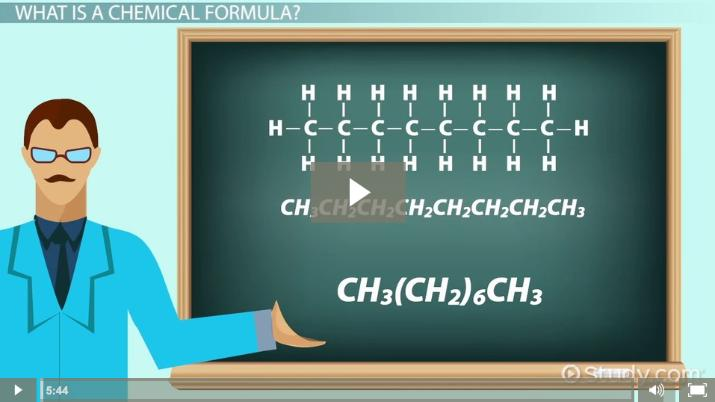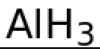# What are Chemical Formulas and How are They Used?

Denise DeCooman, Nissa Garcia, Jamie Lawton
• Author
Denise DeCooman

Denise DeCooman was a teaching assistant for the General Zoology course at California University of Pennsylvania while she earned her Master's of Science in Clinical Mental Health Counseling from fall semester of 2015 and spring of 2017. She also has a Bachelor's of Science in Biological Sciences from California University. She has been writing instructional content for an educational consultant based out of the greater Pittsburgh area since January 2020.

• Instructor
Nissa Garcia

Nissa has a masters degree in chemistry and has taught high school science and college level chemistry.

• Expert Contributor
Jamie Lawton

Jamie has a Ph.D. in Chemistry from Northeastern University and has taught college chemistry.

Learn about chemical formulas. Understand types of chemical formulas such as molecular formula and condensed formula. Discover how to write them and see examples. Updated: 04/08/2022

Show

## What is a Chemical Formula?

Chemicals are found everywhere in nature and all living things are made up of chemicals. All chemicals are composed of elements. What is a chemical formula? A chemical formula is a formula that tells how many atoms of a particular element, or multiple elements, are found within a certain chemical compound. The numbers that appear as subscripts on certain symbols within the formula are used to represent how many of each particular atom are found within the chemical substance. For instance, the chemical formula for water is H2O. The subscript on the symbol for hydrogen is a two, which means there are two hydrogen atoms in water. The oxygen atom lacks a subscript, as there is only one atom of oxygen in the chemical formula for water.

A chemical compound refers to a particular chemical substance that contains atoms of at least two different elements. Molecules, like chemical compounds, contain multiple atoms; however, unlike chemical compounds, a molecule may simply be composed of atoms from the same element. Water is a type of molecule, which consists of two hydrogen atoms and a single oxygen atom. Another example of a molecule is diatomic hydrogen, or {eq}H_{2} {/eq}, which consists of two hydrogens bonded together.An error occurred trying to load this video.

Try refreshing the page, or contact customer support.

Coming up next: What Is a Chemical Property of Matter? - Definition & Examples

### You're on a roll. Keep up the good work!

Replay
Your next lesson will play in 10 seconds
• 0:05 What is a Chemical Formula?
• 1:28 Molecular Formula
• 1:57 Empirical Formula
• 3:06 Structural Formula
• 3:59 Condensed Structural Formula
• 4:43 Lesson Summary
Save Save

Want to watch this again later?

Timeline
Autoplay
Autoplay
Speed Speed

## Chemical Formula Examples

There are four types of commonly observed in chemistry that will be expounded upon in this lesson. Examples of these types of chemical formulas include the:

• Molecular Formula
• Condensed Formula
• Structural Formula
• Empirical Formula

There are many examples of chemical formulas that most high schoolers have witnessed in textbooks or other places. This includes examples such as:

• NaCl (table salt)
• {eq}CH_{4} {/eq}(methane)
• {eq}C_{6}H_{12}O_{6} {/eq} (glucose)
• {eq}C_{3}H_{8} {/eq} (propane)
• {eq}C_{8}H_{18} {/eq} (octane)
• {eq}C_{2}H_{4}O_{2} {/eq} (acetic acid or vinegar)
• {eq}NH_{3} {/eq} (ammonia)
• {eq}C_{6}H_{8}O_{6} {/eq} (ascorbic acid or vitamin C)
• AgCl (silver chloride)
• {eq}H_{3}PO_{4} {/eq} (phosphoric acid)
• {eq}H_{2}SO_{4} {/eq} (sulfuric acid)

### Molecular Formula

What is a molecular formula? A molecular formula is a type of chemical formula that uses symbols of elements from the periodic table and numerical subscripts that reflect the number of atoms of each particular element in the molecular formula. The terms true formula and molecular formula are often used interchangeably. The molecular formula for water is written as {eq}H_{2}O {/eq}, where the number two is written as a subscript that represents that a water molecule is composed of two hydrogen atoms, as mentioned earlier in this lesson. Refer to the image for the molecular formula for the chemical compound aluminum hydride.### Condensed Formula

The condensed formula is a type of chemical formula that contains the symbols for atoms in the order they are observed in the actual chemical substance. The condensed formula is also commonly referred to as the condensed structural formula. The condensed formula is like the structural formula in this way, but the condensed formula differs because it typically omits the line segments which symbolize bonds between the atoms. An example of a condensed formula would be the condensed formula for propane, which is written as {eq}CH_{3}CH_{2}CH_{3} {/eq}. If a condensed structural formula has repeating groups, this will be reflected with another number written outside of parenthesis where the subscript reflects how many times the group repeats. For instance, butane has a molecular formula of {eq}C_{4}H_{10} {/eq}. The condensed formula for butane is {eq}CH_{3}(CH_{2})_{2}CH_{3} {/eq}.

To unlock this lesson you must be a Study.com Member.

## Chemical Formula Activity

A fun and simple way to visualize molecules is to build models. In this activity, you can build models using the information given in the empirical, molecular and structural formulas of different compounds.

What you will need:

• Toothpicks
• Soft colored candies such as gumdrops, sorted by color
• Paper
• Pencil

1) Draw the following table on your paper: (Make sure to leave enough room to fill in the boxes)

 Name Structural Formula Molecular Formula Empirical Formula Ethane Butane Water Hydrogen Peroxide

2) Look up the structural formulas for the three compounds listed above and draw it on your paper.

3) Determine the molecular formula by counting the number of each type of atom. For example, the molecular formula of ethane is C2 H6. Write the molecular formula in the column next to the structural formula of each compound.

4) Next to the molecular formula, write the empirical formula for the compounds by determining the ratios of each type of atom present in the molecule. For example, ethane has 3 hydrogen atoms for each carbon atom, so its empirical formula is CH3.

4) Starting with the empirical formulas, try to build a model of the compounds using the information given in the formula. Select one color candy to represent hydrogen, one color to represent oxygen and one color to represent carbon. You will notice that only the structural formulas give the information required to build a molecule. For the molecular and empirical formulas, you can make a little pile of the required candies.

#### Questions

1) How do the molecular and empirical formulas compare to each other? How are they different?

2) Which formula allows for the best model to be built?

3) When do you think people use each of the types of formulas the most?

4) What are the pros and cons of each formula?

#### What are examples of chemical formulas?

Examples of chemical formulas include H2O (water) and CH4 (methane). Other examples of chemical formulas include AgCl (silver chloride), NaCl (table salt), and C6H12O6 (glucose).

#### What is a basic chemical formula?

A basic chemical formula is a basic form of representation for a chemical compound or molecule. Basic chemical formulas are written using the symbols for elements shown on the periodic table and subscripts which show how many atoms exist in a certain chemical substance.

#### How do you write a chemical formula?

Chemical formulas are written using the symbols of elements from the periodic table which are found in the chemical formula. Subscripts assigned to these elements are written to represent the number of each atom present in the chemical formula.

#### What are the four types of chemical formulas?

Chemical formulas are formulas that show the elements found in a particular chemical substance and how many of each atom is found in that particular chemical substance. The four types of chemical formulas are: molecular, structural, condensed, and empirical.

### Register to view this lesson

Are you a student or a teacher?